# System Matrix

For systems of multiple thick lenses, it is sometimes useful to represent the system by a system matrix. The matrix is built up by multiplying the refraction matrices and translation matrices. The positions of the principal planes, the front and back surface powers, and the equivalent focal length of Gullstrand's equation can be calculated from the system matrix.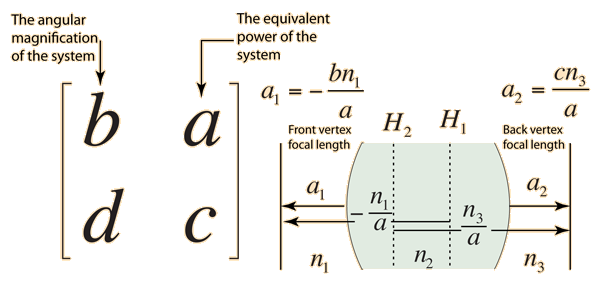This is the form of the system matrix used by Meyer-Arendt. An alternate form is used by Hecht.

 Example calculation of system matrix
 Calculations Alternate form of system matrix: Hecht form
Index

Lens concepts

Thick lens concepts

References
Meyer-Arendt

Hecht

 HyperPhysics***** Light and Vision R Nave
Go Back

# Refraction and Translation Matrices

The system matrix for a thick lens is obtained by multiplying the translation matrix associated with the thickness of the lens times refraction matrix of the first surface and then multiplying by the refraction matrix of the back surface.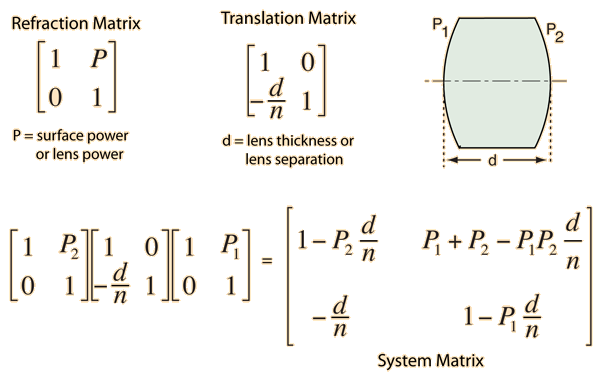Refraction matrix development Translation matrix development
 Alternate form : Hecht form
Index

Lens concepts

Reference
Meyer-Arendt

 HyperPhysics***** Light and Vision R Nave
Go Back

# Refraction Matrix

The use of vergence to describe the refraction at an interface can be used as a starting point for developing a matrix to perform the refraction. Note that the approximations made are valid only for paraxial rays.

The vergence relationship for a surface is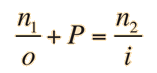and the relevant paraxial approximations are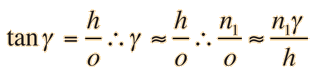These are applied to the refraction at a surface. For more geometrical detail, see surface power.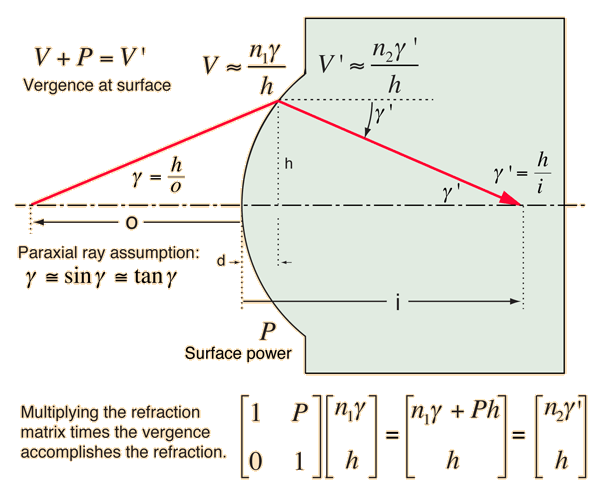Note that the process involves arbitrarily treating the numerator and denominator of the vergence fraction as the elements of a column vector and using matrix multiplication. This is a strategy to represent the refraction as a matrix in such a way that it accomplishes the refraction.

Index

Lens concepts

Reference
Meyer-Arendt

 HyperPhysics***** Light and Vision R Nave
Go Back

# Translation Matrix

The geometry of translation across the thickness of a lens can be put in matrix form for rays satisfying the paraxial approximation.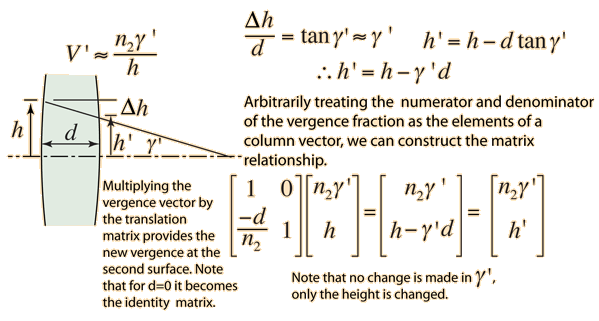Index

Lens concepts

Reference
Meyer-Arendt

 HyperPhysics***** Light and Vision R Nave
Go Back